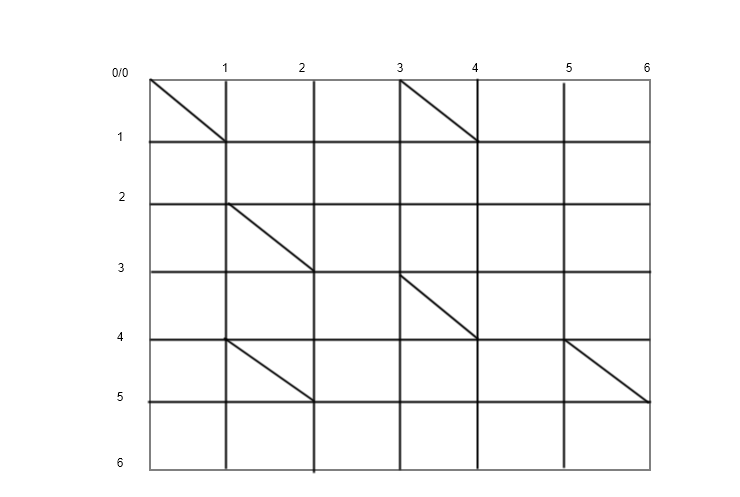# 简述

LCS(Longest common sequence)问题指的是提供两个长度为N的串n和长度为M的串m，求两个串最长的公共子串。其中a是b的子串当且仅当b删除若干个字符后可以得到a，比如"b"是"abc"的子串。

1. 从n中删除一个字符
2. 向n中插入一个字符

LCS问题的经典应用场景有diff和增量计算。

# 解法

## 动态规划

LCS的典型解法就是动态规划。首先我们定义：dp[i][j]=n[0..i]与m[0..j]的最长子串的长度。

• n[i]与m[j]匹配，则此时dp[i][j]=dp[i-1][j-1]+1
• n[i]与m[j]不匹配，此时若二者的最长子串不包含n[i]，则dp[i][j]=dp[i-1][j]
• n[i]与m[j]不匹配，此时若二者的最长子串不包含m[j]，则dp[i][j]=dp[i][j-1]
int lazyDp(int i, int j)
{
if(i < 0 || j < 0)
{
return 0;
}
if(dp[i][j] == -1)
{
dp[i][j] = n[i] == m[j] ? lazyDp(i - 1, j - 1) + 1 : max(lazyDp(i - 1, j), lazyDp(i, j - 1));
}
return dp[i][j];
}


# 贪心算法• 若n[i]与m[j]相同，则建立一条长度为0的边(G[i][j],G[i+1][j+1])。对于若干前后相连的该类边构造的路径，我们称其为蛇。
• 建立长度为1的边(G[i][j],G[i+1][j])
• 建立长度为1的边(G[i][j],G[i][j])

命题1：
（1）偶数号轨迹仅有偶数片段。
（2）奇数号轨迹仅有奇数片段。


命题2：对于轨迹t，其仅有片段{t,t+2,t+4,...}



命题3：对于顶点L(t,d)，其必然是由L(t-1,d-1)向下移动一步或L(t+1,d-1)移动向右移动一步后，并沿着蛇移动到末尾得到的



lcs(n, m)
N = |n|
M = |m|
L = [-M,N] filled with -1
for(d = 0; ; d += 1)
for(t = -d; t <= d; t += 2)
L[t] = max(L[t - 1] + 1, L[t + 1])
//L[t] - x = t -> x = L[t] - t
while(L[t] < N && L[t] - t < M && n[L[t]] == m[L[t] - t])
L[t] += 1
if(L[t] == N && t == N - M)
return d